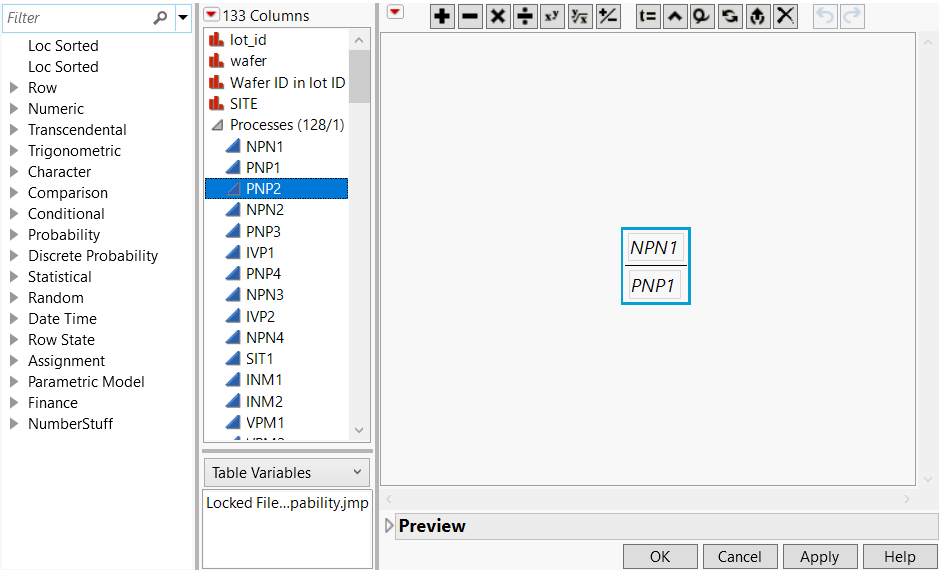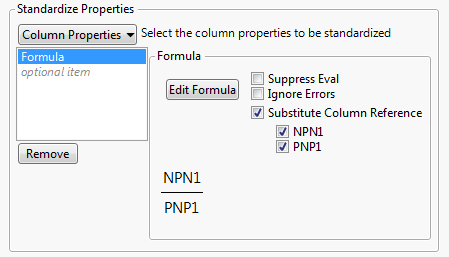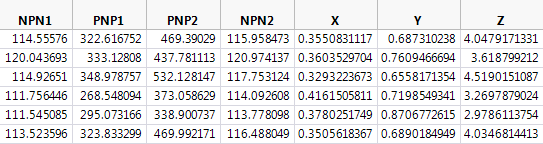Using JMP > Formula Editor > Copy a Formula to Columns on the Right
Publication date: 08/13/2020

# Copy a Formula to Columns on the Right

The Standardize Attributes option in the Cols menu provides a way to replicate a column formula while incrementing each column reference in the formula. This option is an alternative to manually creating new column formulas whose columns increment by one in each of the subsequent columns. For example, if column B contains the formula Ax2, then the standardized column C would contain the formula Bx2, and the standardized column D would contain the formula Cx2.

1. Select Help > Sample Data Library and open Semiconductor Capability.jmp.

2. Select Cols > New Columns.

3. Type 3 next to Number of columns to add and click OK.

4. Select the first new column, select Cols, and then select Formula.

5. Create the formula NPN1/PNP1 and click OK.

Figure 7.11 Create the Formula NPN1/PNP16. Select the three new columns and then select Cols > Standardize Attributes.

7. In the Standardize Properties section, select Formula from the list.

8. Select Substitute Column Reference and leave NPN1 and PNP1 selected.

Figure 7.12 Standardize Properties OptionsNote: In this example, we want to change both NPN1 and PNP1. You can deselect the column that you do not want to change in the subsequent formulas.

9. Click OK.

A formula that is relative to the formula in the first new column is copied to the second and third selected columns.

The formulas in the second and third selected columns increment by one column.

Original formula in the first column: NPN1/PNP1

Relative formula in the second column: PNP1/PNP2

In the data table, PNP2 comes after PNP1.

Relative formula in the third column: PNP2/NPN2

In the data table, NPN2 comes after PNP2.

Figure 7.13 Resulting Data TableNote: Some columns in Semiconductor Capability.jmp were deleted to show the new columns X, Y, and Z in Figure 7.13.

See Example of Standardizing a Formula in the The Column Info Window section for another example.